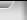By using this website you accept the use of cookies. NOTE: This website is changing soon :-)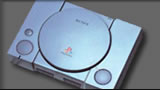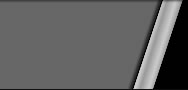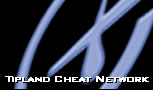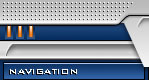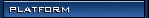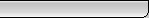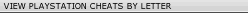[#] [A] [B] [C] [D] [E] [F] [G] [H] [I] [J] [K] [L] [M] [N] [O] [P] [Q] [R] [S] [T] [U] [V] [W] [X] [Y] [Z]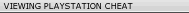Game Name: Agile Warrior Cheats Platform: Viewed: 1883 Date Added: 28/07/2003NOTE: Cheat information below advert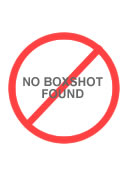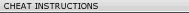Invincibility: LEFT(square x4), UP(triangle x4), RIGHT(circle), DOWN(x), triangle x3, square Max Fuel and Armor LEFT, (Square x 4), UP, (Triangle x 3), RIGHT, (CIRCLE), DOWN,(x) (Triangle x 3), (circle) Max Weapons (999) LEFT, (Square x 4), UP, (Triangle x 3), RIGHT, (CIRCLE), DOWN,(x) (Right1 x 4), (Left1 x 4), (Right2 x 4), (Left2 x 4) B1 Airstrike LEFT, (Square x 4), UP, (Triangle x 3), RIGHT, (CIRCLE), DOWN, ((X) x 6) Mesh Fog Editor LEFT, (Square x 4), UP, (Triangle x 3), RIGHT, (CIRCLE), DOWN,(x) Down, Down, Down, (Triangle x 3) Overhead Map Translucency LEFT, (Square x 4), UP, (Triangle x 3), RIGHT, (CIRCLE), DOWN,(x) (Circle x 5)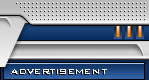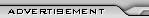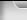Copyright© Tipland Cheat Network 2002 - 2019